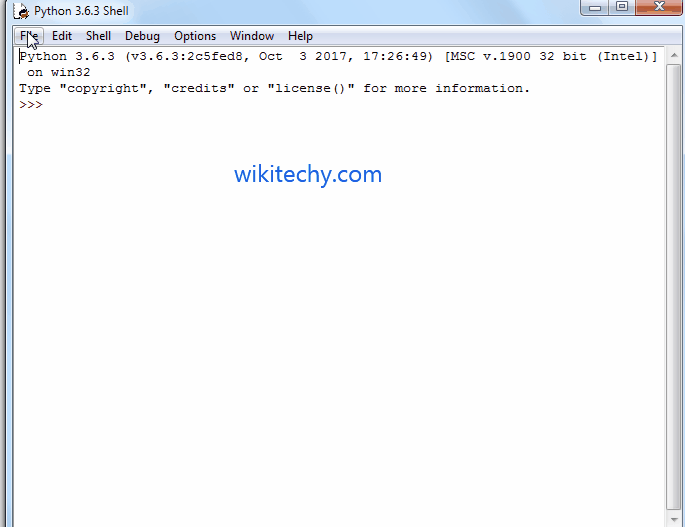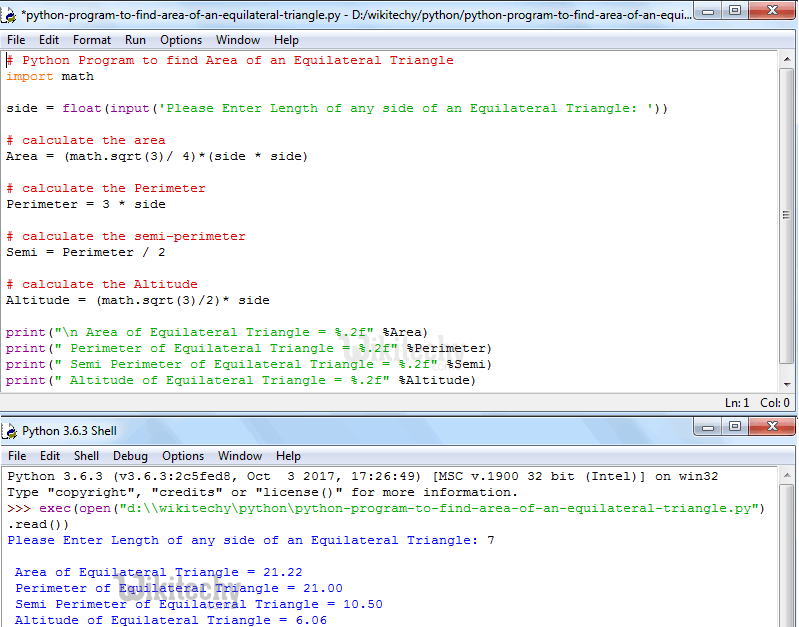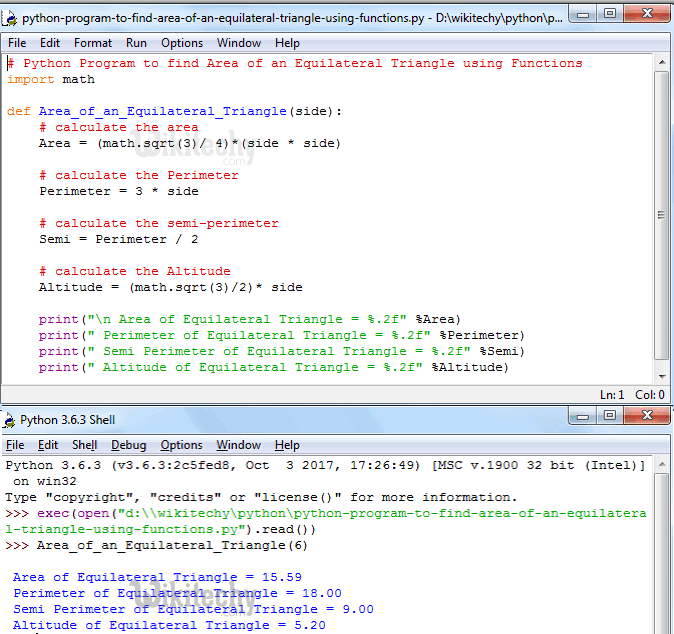# python tutorial - Python Program to find Area of an Equilateral Triangle - learn python - python programming

• To write Python Program to find Area of an Equilateral Triangle, Perimeter, Semi Perimeter and Altitude of a Equilateral Triangle with example.
• Before we step into practical example, Let see the definitions and formulas behind the Area of an Equilateral Triangle.Learn Python - Python tutorial - Python Program to find Area of an Equilateral Triangle - Python examples - Python programs

## Area of an Equilateral Triangle

• The Equilateral Triangle is a triangle with all sides are equal and all of the angles are equal to 60 degrees.
• If we know the side of an Equilateral Triangle then, we can calculate the area of an Equilateral Triangle using below formula.
• Perimeter is the distance around the edges. We can calculate perimeter using below formula:
• We can calculate Semi Perimeter of an Equilateral Triangle using the formula: 3s/2 or we can simply say Perimeter/2.
• We can calculate Altitude of an Equilateral Triangle using the formula: (√3)/2 * s

## Python Program to find Area of an Equilateral Triangle

• This program allows the user to enter length of any one side of an Equilateral Triangle.
• Using this value we will calculate the Area, Perimeter, Semi Perimeter and Altitude of an Equilateral Triangle.

## OutputLearn Python - Python tutorial - Python Program to find Area of an Equilateral Triangle - Python examples - Python programs

## Analysis

• Following statement will allow the User to enter the length of any side in the Equilateral Triangle.
• Next, we are calculating the Area of an Equilateral Triangle using the Formula:
• math.sqrt is the mathematical function, which is used to calculate the square root. It will return error if we miss to use the import math
• In the next line, We are calculating the Perimeter of an Equilateral Triangle using the formula
• In the next line, We are calculating the semi perimeter of an Equilateral Triangle using the following formula.
• We can also find semi perimeter using the standard formula = (3 * side) / 2.
• In the next line, We are calculating the Altitude of an Equilateral Triangle using the formula:
• Following print statements will help us to print the Perimeter, Semi Perimeter, Altitude and Area of an Equilateral Triangle

## Python Program to find Area of an Equilateral Triangle using functions

• This program allows the user to enter length of any one side of an Equilateral Triangle.
• We will pass that value to the function arguments to calculate the area of an equilateral triangle.

## Analysis

• First, We defined the function with one argument using def keyword.
• It means, User will enter any one side of an equilateral triangle.
• Next, We are Calculating the an equilateral triangle as we described in our first example.

## OutputLearn Python - Python tutorial - Python Program to find Area of an Equilateral Triangle using functions - Python examples - Python programs

NOTE: We can call the function with arguments in .py file directly or else we can call it from the python shell. Please don’t forget the function arguments